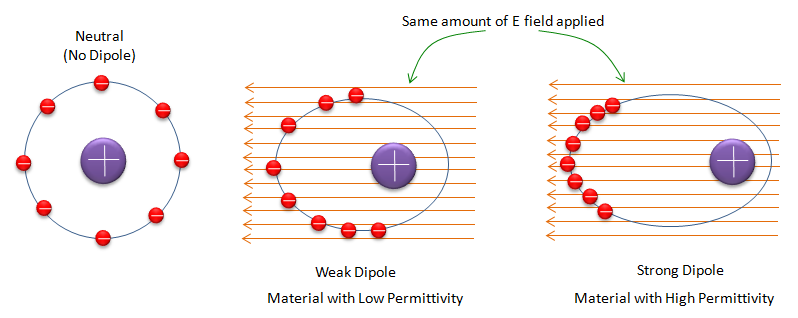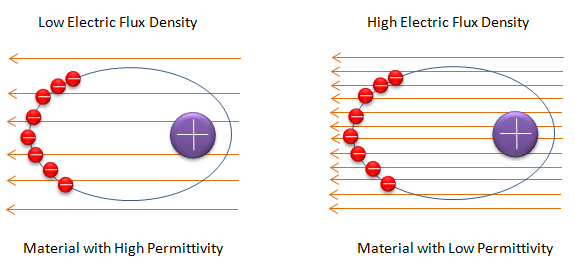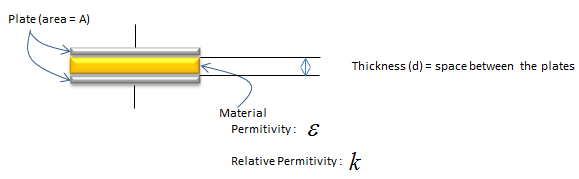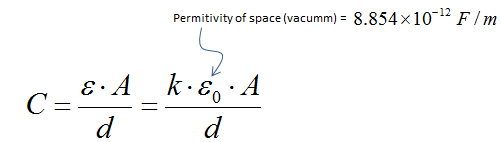RF - Permittivity                                        Home : www.sharetechnote.com

Permittivity is very vague concept.. very hard to grasp the meaning at least to me. But It is very important concept because a lot of other important concepts are derived from this concept.

If you google the meaning of Permittivity, the most common definition would be as follows : Permittivity (Absolute Permittivity) is the measure of the resistance that is encountered when forming an electric field in a medium.

Does this make any sense to you ? At least not much to me.

Whenever you have a difficult concept, one big help would be to come up with an example (real or imaginary). Let's make an imaginary examples as shown below.

The first one (the left one) shows a molecule and it's charge distribution when there is no electric field applied (Don't try argue with illustration of exact charge distribution .. quantom physics ..  I know it is not very accurate illustration). In the first illustration, you see the negative charges are relatively evenly distributed around the positive charge. You can take this first picture as a reference state to compare with other cases (second and third one).

Now let's suppose that electric field is applied through the molecules of two different material (the second and third one).

From the common sense from high school physics, you would guess that negative charges and positive charges would move in opposite directions. (Note : we assume that this materials are dielectric materials and the charges moves but within a certain boundary. In case of conductor, the negative charges (free electrons) gets out of the molecule flows towards the one end).

Due to this movement in opposite direction, you would see the separation (polarization) of charges. But if you compare the second and third one, you see stronger separation in the third one than in the second one. The stronger separation you see, you would say it has higher permittivity.Following is the mathematical expression that explain the exact thing that I mentioned above.Now just "READ" or "Verbalize" the equation above and you would get the intuitive meaning of it.

• Degree of polarization is directly proportional to the electric field applied.
• The stronger the applied electric field, the stronger polarization it shows.
• Permittivity is the proportional coefficient linking Polarization to the applied Electric Field.
• 'Strong Permittivity' means you can achieve the same degree of polarization with less Electric Field.

Let me give you another set of illustrations which has same meaning described above but with a little bit different perspective. If you see the first and the second illustration, you would see the same degree of separation of electric charges (separation of positive and negative charges). Then what is difference ?

In the first illustration, you would see less number of electric field lines than in the second one. It means that less amount of electric field can produce the same degree of polarization.Again, if I express the illustration into a mathematical form, it is as follows.Now just "READ" or "Verbalize" the equation above and you would get the intuitive meaning of it.

• Electric Flux Density is directly proportional to the electric field applied.
• The stronger the applied electric field, the higher Electric Flux Density it gets.
• Permittivity is the proportional coefficient linking Electric Flux Density to the applied Electric Field.
• 'Strong Permittivity' means you can achieve the same Electric Flux Density with less Electric Field.

Permittivity and Capacitance

If you apply a voltage (electric field) on both side of a material, you can create a capacitance. Sometimes you would want to make this kind of capacitor intentionally but sometimes this capacitance would be generated even when you don't want it. Physics doesn't care of your intention, the law applies the same if all the conditions are same.The Capacitance build up by the structure illustrated above can be calculated as follows. (From this formula, you would notice the higher permittiviy, the larger capacitance you will get)Permttivity Table

 Material Relative Permittivity Air 1 Glass (standard) 7.6~8.0 Plexiglass ~2.8 Rubber 2~7 ABS (Plastic) 2.0~3.5 Teflon(PTFE : Polytetrafluoroethylene) 2.1 Mica 3~6 Paper 3.85 FR4 4.7 Oxide 3.9 Diamond 5.5-10 Silicon 11.68